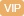| APP | 校园号 | 客服 客服热线：400-863-9889

400-863-9889（2018秋•白云区期末）直接写得数．
（1）8×
 5 6
=
（2）
 2 5
×
 3 5
=
（3）
 3 4
×
 4 9
=
（4）6+
 8 9
=
（5）
 4 15
×
 3 8
=
（6）
 6 11
+2
=
（7）
 2 5
+
 4 7
=
（8）
 4 7
+
 2 3
=
（9）
 3 10
+
 6 5
=
（10）
 2 5
×
 2 3
×
 5 4
=
【考点】分数的加法和减法分数乘法【专题】计算题．

 1 4

【分析】据等性质，方程两边同时加
 1 8
，再同除以
 2 5

 1 4
，再同减2，最后同时除以求；

【解答】解：
 2 5
x-
 1 8
=2，
=8；

 1 4
x÷
 1 4
=2÷
 1 4

 1 4
x=4×
 1 12

x=16.75；

 1 4
（2+8）÷
 1 4
=34÷
 1 4

12.5=25×12，

 7 15
x÷
 7 15
=14÷
 7 15

x9；
 1 4
2+8）=34，

 2 5
x÷
 2 5
=
 17 8
÷
 2 5

+-2=136-2，
x0.4x5.4，

 2 5
x-
 1 8
+
 1 8
=2+
 1 8

 25 x
=
 125 1.2

0.6x÷6=5.40.，
x=30；
x=24．
【点评】题主要考查学运用等式质，及比例基本性质解方程能力．

0/0